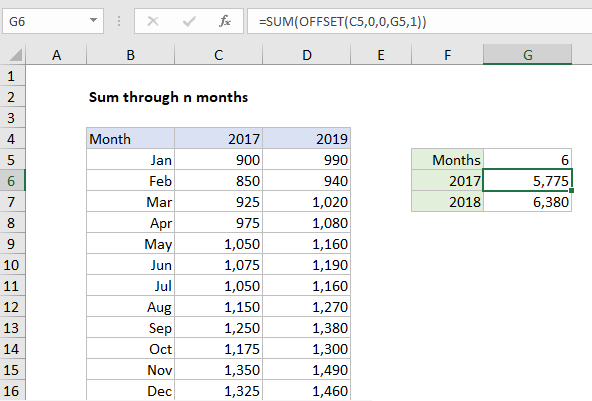## Excel Office

Excel How Tos, Tutorials, Tips & Tricks, Shortcuts

# Sum through n months in Excel

This tutorial shows how to Sum through n months in Excel using the example below;

## Formula

`=SUM(OFFSET(start,0,0,N,1))`## Explanation

To sum a set of monthly data through n number of months, you can use a formula based on the SUM and OFFSET functions. In the example shown, the formula in G6 is:

`=SUM(OFFSET(C5,0,0,G5,1))`

### How this formula works

In the example shown, we have monthly data for the years 2017 and 2018. The goal is to dynamically sum values through a given number of months, hardcoded as 6 in cell G5. This is done by feeding a reference constructed with the OFFSET function into the sum function.

Worked Example:   Sum every n rows in Excel

The OFFSET function let’s you build a reference using a starting point, a row and column offset, and a height and width. OFFSET is handy in formulas that dynamically average or sum “last n values”, “first n values”, and so on. In cell G6, OFFSET is configured like this:

`OFFSET(C5,0,0,G5,1)`

Translated: from a starting position of C5, build a reference 6 rows x 1 column with no offset. With the number 6 in G5, OFFSET returns the reference C5:C10 to SUM:

```=OFFSET(C5,0,0,G5,1) // returns C5:C10
=SUM(C5:C10)) // returns 5775```

The formula in G7 is set up the same way. The only difference is the starting point:

```=OFFSET(D5,0,0,G5,1) // returns D5:D10
=SUM(D5:D10)) // returns 6380```

When the number in G5 is changed, both formulas dynamically update and return a new result.

Worked Example:   Average last 5 values in Excel

### Year to date variation

Instead of hardcoding a value into the formula, you can use the COUNT function to count existing entries in a given column and return that count to OFFSET. A generic version of this formula would look like this:

`=SUM(OFFSET(start,0,0,COUNT(range),1))`

where range references YTD values.

Worked Example:   Count visible rows only with criteria in Excel